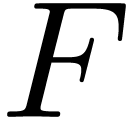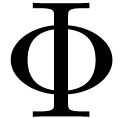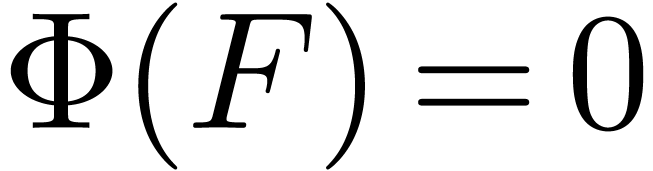From implicit to recursive equations HomepagePublicationsTalksTeXmacsMathemagix

Abstract

The technique of relaxed power series expansion provides an efficient way to solve so called recursive equations of the form, where the unknownis a vector of power series, and where the solution can be obtained as the limit of the sequence. With respect to other techniques, such as Newton's method, two major advantages are its generality and the fact that it takes advantage of possible sparseness of. In this paper, we consider more general implicit equations of the form. Under mild assumptions on such an equation, we will show that it can be rewritten as a recursive equation. Preprint version only: If we are actually computing with analytic functions, then recursive equations also provide a systematic device for the computation of verified error bounds. We will show how to apply our results in this context.

Author: Joris van der Hoeven

Keywords: implicit equation, relaxed power series, algorithm

A.M.S. subject classification: 68W25, 42-04, 68W30, 65G20, 30B10

View: Html, TeXmacs, Pdf, BibTeX

Revisited AAECC Version: Html, TeXmacs, Pdf, BibTeX